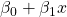# Heteroskedasticity tests

Assume you have a variable y, which has an expectation and a variance. The expectation is often modeled using linear regression so that E(y) equals, on average,. The origin of the variability in y is the residual. Now, standard econometric courses start with the simple notion of “constant variance”, which means that the variance of the disturbances is steady and is not related to any of the explanatory variables that were chosen to model the expectation, this is called homoskedasticity assumption. In fact, in real life it is rarely the case. Courses should start with the heteroskedasticity assumption as this is the prevalent state of the world. In almost any situation you will encounter, the variance of the dependent variable is not constant, it matters what is the x for which we want to determine the variance of y. So:

homoskedasticity assumption: The variance of y is unaffected by the value of x, and so is just a constant number throughout the sample.
heteroskedasticity assumption: The variance of y is affected by the value of x, and so is not a constant number, it changes with the values of x.

Implications
1. Your estimate for the vectorare still unbiased so your point estimation for E(y) are still valid in that sense.
2. Your estimate for the vectorare no longer the most efficient thing you can do. You can get more accurate estimate.
3. Your inference is troubled. Under heteroskedasticity, the confidence intervals are false as they were derived under homoskedasticity.

Testing for it
There are many ways, I give just a couple which are standard practice. I noticed many students learn how to perform the tests but have little idea why these tests work. After reading more than a couple of econometric text books I cannot really fault them as the explanations provided there are somewhat poor.

The two tests are related, the first is the Breusch–Pagan test (named after Trevor Breusch and Adrian Pagan). The second is White test. I read somewhere that white’s paper from 1980 is the most cited paper in economics, which points to the pervasive nature of the problem. We use the squared residual from the regression, denote itas an estimate for the variance. The reason is: V(residual) = E(residual^2) – E(residual)^2 = E(residual^2) – 0^2. So that is whyis used to measure the variance of y. The idea is simple, we perform an extra regression called auxiliary regression:and check if the regression is meaningful, that is, if one of the covariates is related to the variance of y. The statistic isdistributed and is computed as (number of observations)*(R^2 of the auxiliary regression).

White test is only slightly different, we add the squares of the covariates and the cross product to check for a non-linear impact on the variance. The computation of the test statistic is the same.

Demonstration
We will use three variables, unemployment rate, Short rate and Industrial production. Data is public, you can just copy paste the code to load it.Transform to stationary to avoid spurious regression,

A regression:

The Breusch–Pagan test – using a built-in function:

The Breusch–Pagan test – just so you know what you are doing:

We can see the variable that is related to the variance is Industrial Production, or is it?..

White test – no built in function that I know of.

Here we see that the IP-squared.residual relation is less obvious. The variance of the dependent variable has non-linear relation with the variable Industrial Production as you can see in the following table:

Estimate Std. Error t.value P.val
(Intercept) 0.0248 0.0021 11.94 0.0000
IP -0.0070 0.0043 -1.62 0.1048
ShortRate 0.0018 0.0044 0.41 0.6833
I(IP^2) 0.0104 0.0027 3.79 0.0002
I(ShortRate^2) -0.0011 0.0028 -0.37 0.7084
I(IP * ShortRate) 0.0148 0.0100 1.48 0.1404

How to deal with it?
Weighted Least Squares (WLS) is a reasonable solution. We scale the equations so that observations with less information in them (high residuals) get lower weights. How much lower? proportional to their variance (squared residual) or to their standard deviation. Theoretically, if we knew the exact true variances, then WLS is better. However, we have one observation as an estimate of the variance, the estimated residual of each observation, and that residual is often a poor estimate for the unknown true residual which depend on the true generating process. So apart from the fact that you have only one, it is more often than not inaccurate estimate since you probably have not considered the “right” explanatory variables or the equation itself is not even linear. For those reasons, WLS is not as popular in practice as you might expect from the theory.
In R, it is very easy to do using the argument “weights” as follows:To leave you with something to think about, does the regression resulting from the WLS procedure is better fit or worse fit in terms of R^2? higher R^2 or lower R^2 than the original regression with equal weights for all observation? (worse fit, but why?)